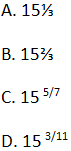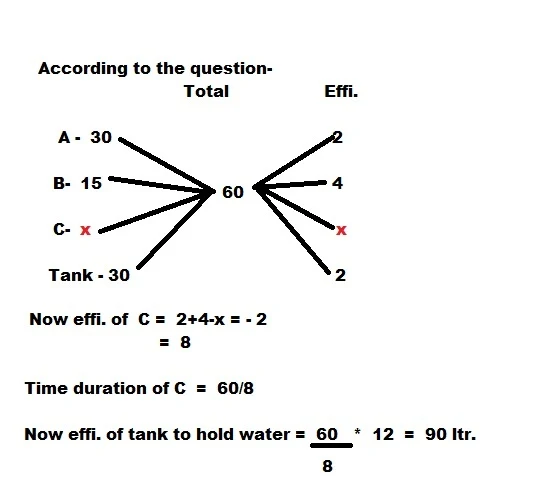Gurpurab Offer - Use Code GURPURAB2023

# Time and Work Quiz for IBPS PO: Part 1

1. A work could be completed by certain workers in 22 days. However, due to 3 workers being absent, it was completed in 24 days. The original number of workers was -
A. 33
B. 18
C. 36
D. 25

2.A and B together can do a piece of work in 18 days, B and C in 24 days, and A and C in 36 days. The number of days, all of them working together take to complete the work, is-
A. 18
B. 16
C. 17
D. 15

3. Two taps can fill a tank in 4 hours and 5 hours respectively. It both the taps are opened alternatively for 1 hour, starting with the first. How much time will it take to all the tank.
A. 4 h 20 m
B. 4 h 24 m
C. 4 h 30 m
D. 4 h 36 m

4. Twenty women can do a work in sixteen days. Sixteen men can complete the same work in fifteen days. The ratio between the capacity of a man and a woman is:
A. 3 : 4
B. 4 : 3
C. 5 : 3
D. 5 : 7

5. 5 women and 2 men working together can do four times as much work per hour as a woman and a man together. Compare the work of a woman with that of a man.
A. 3 : 2
B. 1 : 2
C. 2 : 1
D. 2 : 3

6. 12 boys or 15 girls can do a certain work in 14 days. Find the number of days that 7 boys and 5 girls will take to complete the same work.7. Two pipes A and B can fill a cistern in 20 and 24 min respectively. Both pipe being opened; find when the first pipe must be turned off, so that the cistern may be filled in 12 min.
A. after 25 minutes
B. after 10 minutes
C. after 15 minutes
D. after 20 minutes

8. Pipes A and B fill a tank in 30 minutes and 15 minutes. Pipe C drains 12 litres of water in a minute. If all of them are kept open when the tank is full, the tank empties in 30 minutes. How much water can the tank hold?
A. 80 litres
B. 90 litres
C. 75 litres
D. None of these

9. 10 boys can finish a certain work in 10 days, whereas it takes 12 girls to finish it in 10 days. If 15 boys and 6 girls undertake to complete the work, how many days will they take to complete it?
A. 9 days
B. 7 days
C. 5 days
D. 3 daysE.

10. A can do a piece of work in 10 days and B can do it in 15 days. They start the work together but B goes away after 3 days and A continues till the work is-
A. 10 days
B. 8 days
C. 11 days
D. 11.5 days

#### 1. Option A.

Let x = original number of workers
So, ATQ, 22x = 24(x – 3)
or, 22x = 24x – 72
⇒ x = 36

#### 2. Option B.

The number of days taken by A, B and C to complete the work while working together-#### 3. Option (B)

Assuming Capacity of Tank=4*5=20L
Tap 1 = 20/4 =5L/h
Tap 2 = 20/5= 4L/h
After 4 hours ,total filled=5+4+5+4=18L
Time taken for Remaining=2/5hours =24min
So. Total time= 4h 24min

20W×16=16M×15
Man/Woman=4/3

#### 5. Option (C)

(5W+2M)’s 1 day work= (1W+1M)’s 4 Day’s Work
5W+2M=4W+4M
W/M =2/1

#### 6. Option (D)

No. of Days= 1/(7/14*12 + 5/14*15) = 15 3/11 Days

#### 7. Option (B)

The Cistern is to be filled in 12 min. Second pipe can fill only 12/24=1/2 of the cistern in total time.
This means the other half of the tank will be filled in 10 min.

#### 8. Option (B)10B*10=12G*10
5B=6G
(15B+6G)X=10B*10
20B.X=10B*10
X=5 days

#### 10. Option (B)